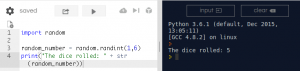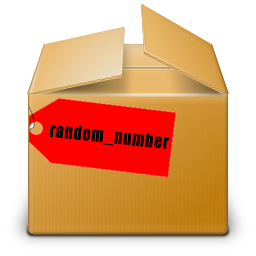# Python : Roll the Dice

This short exercise will cover topics relevant to GCSE computing including:

• Variables
• Data Types
• Random Numbers
2. Create a ‘New Repl’3. Then choose ‘Python 3’ as the language
4. Type the following code into the left pane:``` import random random_number = random.randint(1,6) print("The dice rolled: " + str(random_number))```
5. Then click the ‘run’ button at the top several times and notice how the output changes randomly.

You should see the output of your code in the dark panel on the left:• Line 1 imports code that someone else has written. This saves us time as now we don’t need to write lots of code to generate random numbers. As you write more complex programs you will import lots of other code.
• Line 2 creates a variable called ‘random_number’ then uses a ‘function’ called ‘randint’ (contained in the imported ‘random’ code ) to generate a random integer (or whole number with no decimal points)
• Line 3 uses ‘print’ to say display the random number on the screen. Notice that the ‘Data Type’ for the variable ‘random_number’ is an integer (number) and we need to convert it to a string (text) for displaying on the screen.

## Terms:

• Variable – This time we used a ‘variable’ (or storage box) called ‘random_number’ and we put a number inside it.• Data Type – variables can be numbers or text. We need to use the correct data type or we may create bugs. Imagine asking the computer to multiply 3 by John or even multiply 3 by seven. In both cases the computer will be confused as it can’t multiply a number by some text – so we need to tell the computer the ‘Data Type’ we expect to use. In the exercise we wanted to print the random number but we needed to convert (or cast) it as a string/text first.
• Output – We used a special command called ‘print’ to show output on the screen
• Functions – In computing we usually call special commands like ‘randint’ and ‘print’ functions.
• Import – We do not want to re-invent the wheel so we import modules of code written by other people. In this program we imported code that helps us create random numbers

## Next:

Try Roll the Dice Multiple Times next.